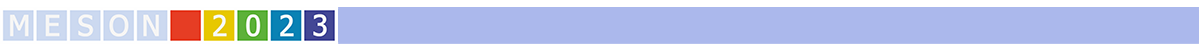#MESON2023

Jun 22 – 27, 2023
Auditorium Maximum
Europe/Warsaw timezone

## Properties of the Tcc(3875) and its heavy-quark spin partner in nuclear matter

Jun 22, 2023, 5:15 PM
20m
Medium lecture hall (A) (Auditorium Maximum)

Parallel

### Speaker

Víctor Montesinos Llácer (IFIC)

### Description

We discuss the modification of the properties of the tetraquark-like $T_{cc}^+(3875)$ in dense nuclear matter. We consider the $T_{cc}^+$ in vacuum as a purely molecular isoscalar ($D^0D^{*+}/D^+D^{*0}$) bound state in $S-$wave, generated from a heavy-quark symmetry leading-order interaction between the charmed mesons. We compute the $D$ and $D^*$ spectral functions embeded in a nuclear medium and use them to determine the corresponding $T_{cc}^+$ self energy and spectral function. We find important modifications of the $DD^*$ scattering amplitude and of the pole position of the $T_{cc}^+$ exotic state already for $\rho_0/2$, with $\rho_0$ the normal nuclear density. We also discuss the dependence of these results on the $D D^*$ molecular component in the $T_{cc}^+$ wave-function. Finally, we perform a similar analysis for the isoscalar $J^P=1^+$ heavy-quark spin symmetry partner of the $T_{cc}^+$ ($T_{cc}^{*+}$)
by considering the $D^{*0}D^{*+}$ scattering $T-$matrix.

### Primary authors

Víctor Montesinos Llácer (IFIC) Miguel Albaladejo (JLab) Laura Tolos (ICE, Barcelona) Juan Miguel Nieves Pamplona (IFIC)